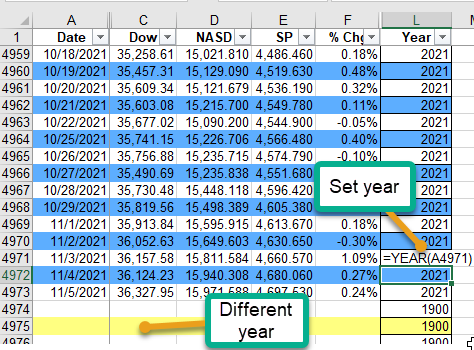# Excel VBA

## Color Rows by Criteria

I have a spreadsheet where the closing value of my portfolio, the NASDAQ, the DJIA, and the S&P close is recorded. See sample below.

Since it is almost 5K rows, I decided I wanted to color the rows with a specific color based on the year.

My list is complete from year 2000 to date (except for a short period where I lost access to some files in a move to new PC).

```Sub ReColorSnapShot()
'ARB 12/02/2020
'+---------Unlock SNAPSHOT,set color scheme by Year, close and report--------+
'+---------------------------------------------------------------------------+
Dim Ranger  As String
Dim LastCol As Long, lastRow As Long, ColorNum As Long
Dim SortRanger As Variant
Dim C   As Long
Dim H   As Long
Dim Quo As String
Dim YearNm As Variant, RGBnm As Variant 'Arrays
Quo = Chr(34)   'Quotes character
Application.ScreenUpdating = True
Sheets("SnapShot").Activate
ActiveSheet.Unprotect
lastRow = Range("L99999").End(xlUp).Row
LastCol = 12    'Last column set in stone; other things past there
Range(Cells(2, 1), Cells(lastRow, LastCol)).Select
Selection.Interior.ColorIndex = False   'Remove interior color from all
Cells(2, 1).Select
YearNm = Array("2000", "2001", "2002", "2003", "2004", "2005", "2006", _
"2010", "2011", "2012", "2013", "2020", "2021", _
"2007", "2008", "2009", "2014", "2015", _
"2016", "2017", "2018", "2019", "2022", _
"2023", "2024", "1900") 'chgd 1900 to light yellow 03/16/2021
'Only have daily data after 4/15/2000
RGBnm = Array(RGB(153, 204, 255), _
RGB(131, 241, 123), _
RGB(255, 153, 255), _
RGB(255, 255, 0), _
RGB(250, 191, 143), _
RGB(205, 216, 176), _
RGB(153, 204, 0), RGB(204, 255, 204), RGB(102, 204, 255), _
RGB(255, 204, 153), RGB(204, 192, 218), _
RGB(207, 183, 255), RGB(93, 174, 255), RGB(255, 128, 128), _
RGB(255, 204, 0), RGB(192, 192, 192), RGB(255, 255, 204), _
RGB(204, 204, 255), RGB(204, 255, 255), RGB(255, 102, 0), RGB(115, 120, 115), _
RGB(223, 184, 223), RGB(200, 181, 124), RGB(248, 54, 123), RGB(28, 155, 147), _
RGB(255, 255, 129)) '25'    'Array of color schemes
For H = 0 To UBound(YearNm) 'Step thru years
SortRanger = RGBnm(H)
For C = 2 To lastRow
Application.StatusBar = YearNm(H)   'Show year progress
If Cells(C, LastCol).Text = YearNm(H) Then
If C Mod 2 = 0 Then     'Determine if even or odd numbered row
Sheets("SnapShot").Range(Cells(C, 1), Cells(C, LastCol)) _
.Interior.Color = SortRanger    'Year Color
Else
Sheets("SnapShot").Range(Cells(C, 1), Cells(C, LastCol)) _
.Interior.ColorIndex = False    'No color
End If
End If
Next C
Next H
Application.StatusBar = False
Cells(C - 10, 1).Select
ActiveSheet.Protect

YearNm = 0
RGBnm = 0
C = 0
MsgBox "Updated Snapshot Colors", vbInformation, "Done" ' Moved 10/29/21'
End Sub
```

In this example some years do not yet have an assigned color. Some of the programming got a little messy. All in all, the initial coding took about an hour and debugging another hour or so.